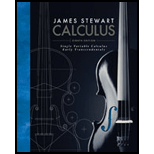Chapter 11, Problem 22RE### Single Variable Calculus: Early Tr...

8th Edition
James Stewart
ISBN: 9781305270336

#### Solutions

Chapter
Section### Single Variable Calculus: Early Tr...

8th Edition
James Stewart
ISBN: 9781305270336
Textbook Problem

# Determine whether the series is convergent or divergent.22. ∑ n = 1 ∞ n + 1 − n − 1 n

To determine

Whether the series is convergent or divergent.

Explanation

Result used: The Limit Comparison Test

(1) “Suppose that an and bn are the series with positive terms, if limnanbn=c , where c is a finite number and c>0 , then either both series converge or both diverge.”

(2) The p-series is convergent if p>1 and divergent if p1 .

Calculation:

The given series n=1an=n=1n+1n1n

n+1n1n=n+1n1n×n+1+n1n+1+n1=(n+1)2(n1)2nn+1+n1=(n+1n+1)nn+1+n1=2nn+1+n1

Simplify further as follows,

n21<n2n21<2n2n21<4n2n2n21<4n(n+1)+(n1)

Take square root on both sides,

(n+1)+(n1)+2n21<4n(n+1+n1)2<(2n)2n+1+n1<2n1nn<2nn+1+n1   (Divide by n)

Consider the series n=1an=n=12n(n+1+n1) is greater than n=1bn=n=11nn

### Still sussing out bartleby?

Check out a sample textbook solution.

See a sample solution

#### The Solution to Your Study Problems

Bartleby provides explanations to thousands of textbook problems written by our experts, many with advanced degrees!

Get Started

## Additional Math Solutions

#### Find more solutions based on key concepts